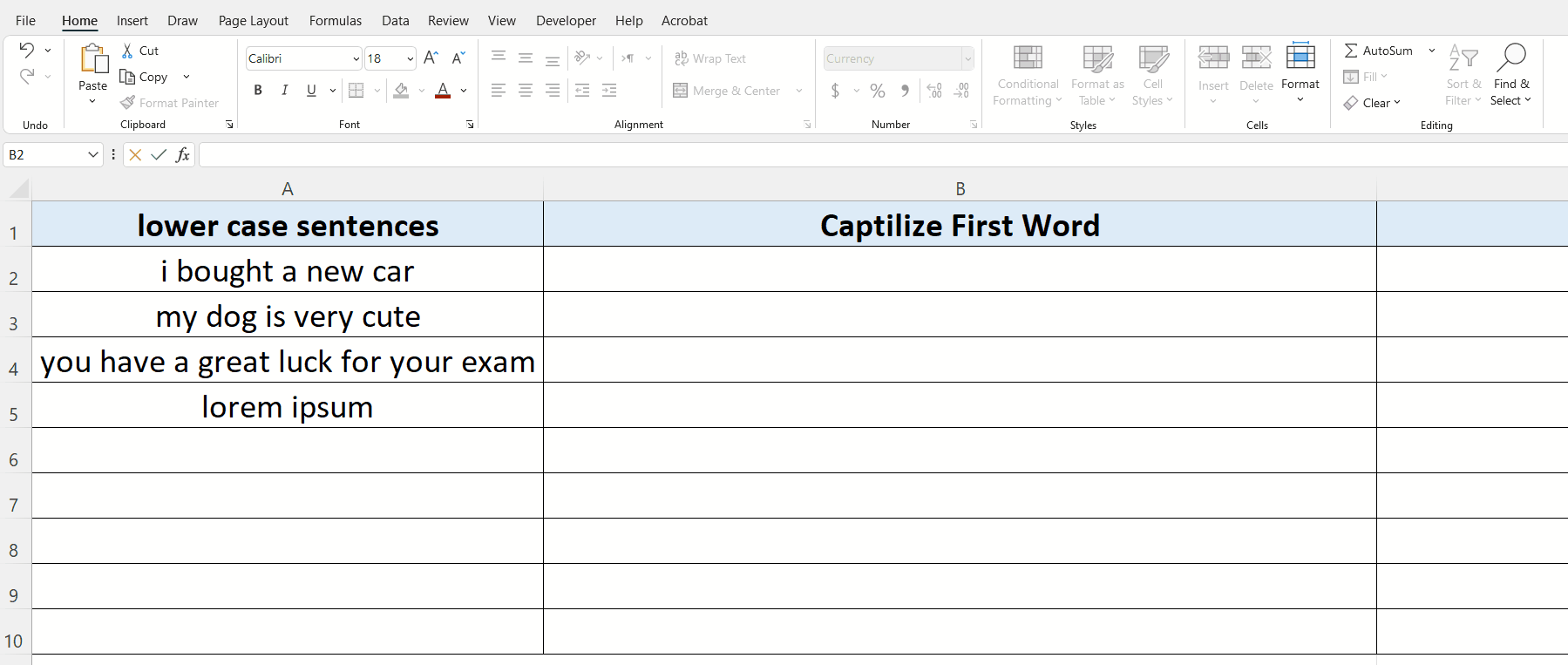# How to capitalize the first letter of each word in Excel

In this tutorial we’ll learn how to capitalise the first letter of each word in Excel by using a very simple built-in function of Excel. Let’s learn how to do it by following the step below.When we hear the word Microsoft Excel, most definitely we think about doing some data analysis and performing some mathematical calculations. However, Excel provides some very simple and easy to use methods to be operated upon text data as well.

### Step 1 – Implement the formula in a suitable cell– If you already have a data set for which you wish to capitalise the first letter of each word then use the following formula

=PROPER(text)

– Implement this formula in the cell next to the cell containing the original text and then drag down the formula to the last row of the data set as shown in the figure above.

So this is how Excel can save us from rewriting all the sentences again and by using a simple formula all text is converted in the desired format.